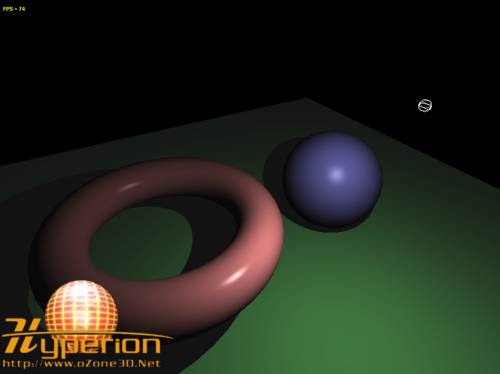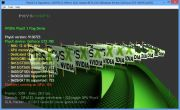Home Utilities Tutorials 3D Demos Graphics Cards Forums About

 GeeXLab
Current version: 0.45.1
>GeeXLab homepage

 FurMark
Current version: 1.30.0
>FurMark homepage

 GPU Caps Viewer
Current version: 1.55.0.0
>GPU Caps Viewer homepage

 GPU Shark
Current version: 0.26.0.0
>GPU Shark homepage

 Blogs
>JeGX's HackLab

 Geeks3D's Articles
>GPU Memory Speed Demystified

>How to Get your Multi-core CPU Busy at 100%

>How To Make a VGA Dummy Plug

>Night Vision Post Processing Filter

 PhysX FluidMark
Current version: 1.5.4
>FluidMark homepage

 TessMark
Current version: 0.3.0
>TessMark homepage

Current version: 0.3.0

 Demoniak3D
Current Version: 1.23.0
>Demoniak3D
>Libraries and Plugins
>Demos
>Codes Samples

3D Graphics Search Engine:Lighting with GLSL
Phong Model

By Jérôme GUINOT aka 'JeGX' - jegx [at] ozone3d [dot] net

Initial draft: February 19, 2006
Update: March 8, 2006

[ Index ]

4 - Lighting AttenuationLighting equation coefficients that are dependent on a specific source of light can be optionally multiplied by an attenuation factor. This attenuation factor allows to simulate the light fading with distance.

`If = (As*Am) + ((Al*Am) + Id + Is) * att`

There are several ways to calculate the attenuation factor. We're going to see two of them.

## 4.1 - The standard method

This method exploits OpenGL coefficients of each light in GLSL:
- gl_LightSource.constantAttenuation or Kc
- gl_LightSource.linearAttenuation or Kl

From these coefficients, the attenuation factor can be obtained by:

att = 1.0 / (Kc + Kl*d + Kq*d2)

where d stands for the distance between the position of the light and the currenlty processed vertex:

```lightDir = vec3(gl_LightSource.position.xyz - vVertex);
float d = lenght(lightDir);```

In OpenGL terms, attenuation factors are defined by:

```glLightf( GL_LIGHT0, GL_CONSTANT_ATTENUATION, 0.0f );
glLightf( GL_LIGHT0, GL_LINEAR_ATTENUATION , 0.0f );
glLightf( GL_LIGHT0, GL_QUADRATIC_ATTENUATION , 0.0002 );```

And in Demoniak3D, these factors are defined in the light node:

```<light name="Light_01"

Here is now our attenuated point light vertex shader:

```[Vertex_Shader]

varying vec3 normal, lightDir, eyeVec;
varying float att;

void main()
{
normal = gl_NormalMatrix * gl_Normal;

vec3 vVertex = vec3(gl_ModelViewMatrix * gl_Vertex);
lightDir = vec3(gl_LightSource.position.xyz - vVertex);
eyeVec = -vVertex;

float d = length(lightDir);

att = 1.0 / ( gl_LightSource.constantAttenuation +
(gl_LightSource.linearAttenuation*d) +

gl_Position = ftransform();
}```

And here is our attenuated point light pixel shader:

```[Pixel_Shader]

varying vec3 normal, lightDir, eyeVec;
varying float att;

void main (void)
{
vec4 final_color =
(gl_FrontLightModelProduct.sceneColor * gl_FrontMaterial.ambient) +
(gl_LightSource.ambient * gl_FrontMaterial.ambient)*att;

vec3 N = normalize(normal);
vec3 L = normalize(lightDir);

float lambertTerm = dot(N,L);

if(lambertTerm > 0.0)
{
final_color += gl_LightSource.diffuse *
gl_FrontMaterial.diffuse *
lambertTerm * att;

vec3 E = normalize(eyeVec);
vec3 R = reflect(-L, N);

float specular = pow( max(dot(R, E), 0.0),
gl_FrontMaterial.shininess );

final_color += gl_LightSource.specular *
gl_FrontMaterial.specular * specular * att;
}

gl_FragColor = final_color;
}```

The attenuation factor is computed in the vertex shader and then is passed to the pixel shader through the varying float att; variable.Fig.5 - The point_light_att.xml demo.

This method uses an imaginary sphere in which the light lies in. This sphere represents the light's influence area (i.e the farthest distance an object can be influenced by the light). The attenuation factor decreases along the radius, and becomes equal to zero on the edge of and outside the sphere. This factor is calculated in the pixel shader and assumes that a variable holding the inverse of the radius of the sphere is defined (in order to avoid a division):

```float distSqr = dot(lightDir, lightDir);
float att = clamp(1.0 - invRadius * sqrt(distSqr), 0.0, 1.0);
vec3 L = lightDir * inversesqrt(distSqr);```

This piece of code needs to be explained. The norm of the lightDir vector, is given by:

dist = sqrt(lightDir.x2 + lightDir.y2 + lightDir.z2)

This norm can be written using a dot product:

dist = sqrt( dot(lightDir, lightDir) )
and
distSqr = dot(lightDir, lightDir)

distSqr and invRadius allow to calculate the attenuation factor. The L vector, that is the normalized direction vector, can be obtenaid by the usual normalization formula:

magnitude: |r| = sqrt( x2 + y2 + z2 )
magnitude: |r| = sqrt( distSqr )

unit vector u: u = (1/|r|) * U
unit vector u: u = inversesqrt(distSqr) * U

The final word: all vertex / pixel shaders previously seen are for all-purposes (i.e they are not bound to Demoniak3D), do not take input parameters (i.e no uniform variables) and so they can be effortless used in any OpenGL application. That said, have a good coding!

5 - Further ResourcesDemoniak3D Phong Lighting Source CodesUpdate: February 19, 2006All projects have been successfully tested on a nVidia Geforce 7800GT (with Forceware 81.98) and on an ATI Radeon X700 Pro (with Catalyst 6.1).All projects have been successfully tested on the flollowing configurations: nVidia Geforce 7800GT - Forceware 81.98 ATI Radeon X700 Pro - Catalyst 6.1 ATI Radeon 9800 Pro - Catalyst 6.2 *** These source codes require Demoniak3D to work properly. Demoniak3D is available here: [:: Demoniak3D Demo-System ::].

[ Index ]

 GeeXLab demosGLSL - Mesh exploderPhysX 3 cloth demoNormal visualizer with GS